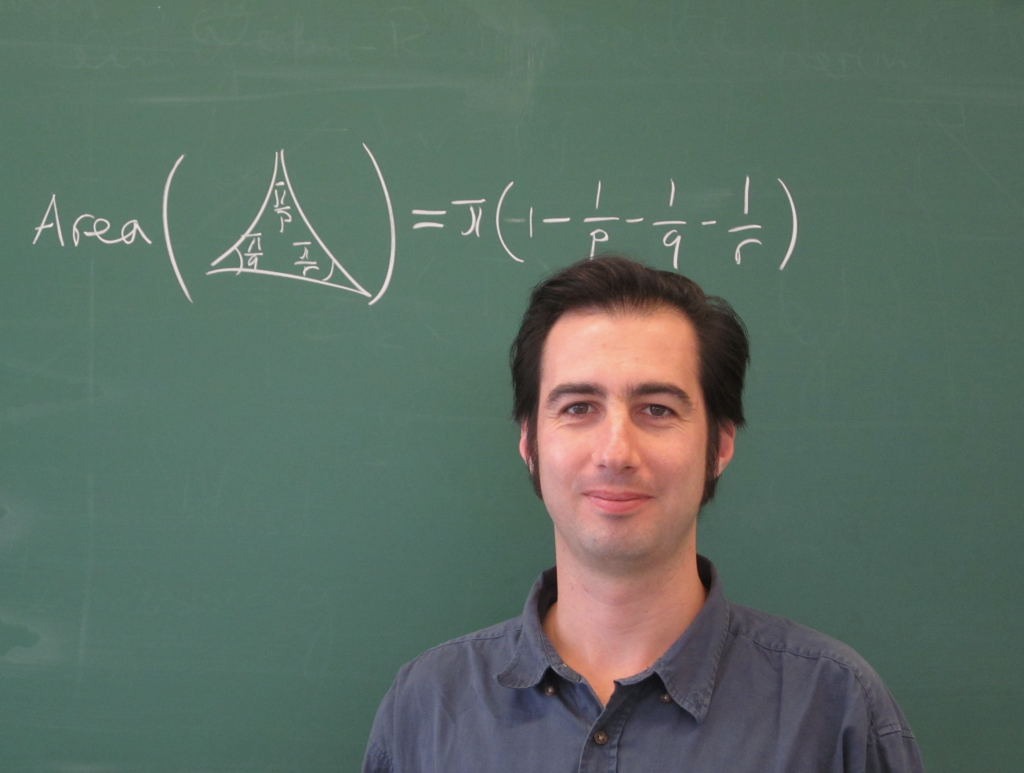## ICMS Seminar

14.11.23, 16. 11.23, 21.11.23 & 23.11.23
14:00, Sofia time

ICMS-Sofia, Room 403# Invariant theory, homogeneous projective varieties, and momentum maps

Let $f:K\to U(V)$ be a unitary representation of a compact Lie group $K$ with Lie algebra $\mathfrak k$. This series of talks will be devoted to some recent developments based on relations between the following three classical notions.

• The ring of $K$-invariant polynomials $\mathbb C[V]^K$ is finitely generated by homogeneous elements, by a theorem of Hilbert, but the proof is notoriously nonconstructive. The degrees of a minimal set of generators are canonically determined by $f$, and will be the focus of the discussion.
• The $K$-equivariant momentum map $\mu:\mathbb P(V)\to \mathfrak k^*$ associated to the Fubini-Study form encodes in its image properties of the invariant ring $\mathbb C[V]^K$ and the $K$-module structure of the entire polynomial ring $\mathbb C[V]$, due to theorems of Mumford and Brion. While many general properties of momentum images have been established, explicit descriptions remain unknown in many cases south for in physics and quantum information theory.
• Projective geometry of complex $K$-orbits in $\mathbb P(V)$; their fundamental forms, osculating varieties and secant varieties.

General relations between the first two topics are well established, even in a more general context. There are also well known relations between invariant theory and projective geometry, but secant varieties have only recently been brought to the discussion and are subject of current interest.

After introducing the basic notions, I will derive some properties of momentum images related to fundamental forms and osculating varieties, as well as a lower bound on the minimal positive degree of a homogeneous invariant, derived using secant varieties. At the end I will present a class of homogeneous projective varieties, characterized by a special property of their secant varieties, where the relations between the above three concepts take a particularly pristine form.